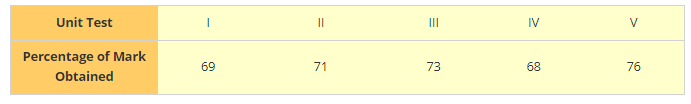# The percentage of marks obtained by a student in the monthly unit tests are given below:

Question:

The percentage of marks obtained by a student in the monthly unit tests are given below:Find the probability that the student gets

1. More than 70% marks

2. Less than 70% marks

3. A distinction

Solution:

1: Let E be the event of getting more than 70% marks

No of times E happens = 3

Probability (Getting more than 70\%) $=\frac{\text { Number of times student got more than 70 }}{\text { Total no of exams taken }}$

= 3/5 = 0.6

2. Let F be the event of getting less than 70% marks

No of times F happen = 2

Probability(Getting less than $70 \%)=\frac{\text { Number of times student got less than } 70}{\text { Total no of exams taken }}$

= 2/5 = 0.4

3. Let G be the event of getting distinction

No of times G happen = 1

Probability(Getting distinction) $=\frac{\text { Number of times student got distinction }}{\text { Total no of exams taken }}$

=1/5 = 0.2